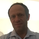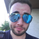22974 views
22974
Stochastic Momentum Index (SMI) or Stoch MTM is used to find oversold and overbought zones. It also helps to figureout whether to enter short trade or long trade.

Red Shade in the Top indicates that the stock is oversold and the Green shade in the bottom indicates overbought.

Strategy:

Enter Long once the Overbought Zone ended and there's a crossover below -35.
Exit Long once the oversold zone is ended and there's a crossover.

Enter Short once the oversold zone is ended and there's a crossover above 35.
Exit Short once the Overbought Zone ended and there's a crossover.

Backup: Always use with another indicator because there will be multiple up and down movement in one Trend.
```//@version=2
//Stochastic Momentum Index
// Author: Surjith S M (India)
//Thanks UCSgears, lonestar108
study("Stochastics Momentum Index", shorttitle = "Stoch_MTM")
a = input(10, "Percent K Length")
b = input(3, "Percent D Length")
ob = input(40, "Overbought")
os = input(-40, "Oversold")
// Range Calculation
ll = lowest (low, a)
hh = highest (high, a)
diff = hh - ll
rdiff = close - (hh+ll)/2

avgrel = ema(ema(rdiff,b),b)
avgdiff = ema(ema(diff,b),b)
// SMI calculations
SMI = avgdiff != 0 ? (avgrel/(avgdiff/2)*100) : 0
SMIsignal = ema(SMI,b)
emasignal = ema(SMI, 10)

h0 = hline(40)
h1 = hline(-40)

//Color Definition for Stochastic Line
//col = SMI >= 40 ? green : SMI <= -40 ? red : black

plot(SMIsignal, title="Stochastic", style=line, color=black)

plot(emasignal, title="EMA", style=line, color=red)

level_40 = 40
level_40smi = SMIsignal > level_40 ? SMIsignal : level_40

level_m40 = -40
level_m40smi = SMIsignal < level_m40 ? SMIsignal : level_m40

p1 = plot(level_40)
p2 = plot(level_40smi)

p3 = plot(level_m40)
p4 = plot(level_m40smi)

fill(p1, p2, color=red, transp=40, title='OverSold')

fill(p3, p4, color=green, transp=40, title='OverBought')

```